### Home > CALC > Chapter 6 > Lesson 6.2.1 > Problem6-64

6-64.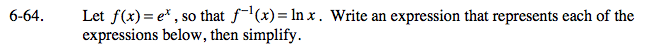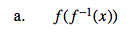Do you remember the definition of inverse functions?
f(x) and g(x) are inverses if f(g(x)) = x...
Well, f(f −1(x)) = elnx = ______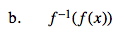Refer to the hint in part (a).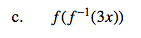Refer to the hint in part (a).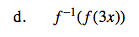Let 3x = U.